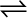7.14 One mole of H2O and one mole of CO are taken in 10 L vessel and heated to
72
5 K. At equilibrium 40% of water (by mass) reacts with CO according to the equation,

H2O (g) + CO (g)H2 (g) + CO2 (g)

Calculate the equilibrium constant for the reaction.

The given reaction is:
Therefore, the equilibrium constant for the reaction,